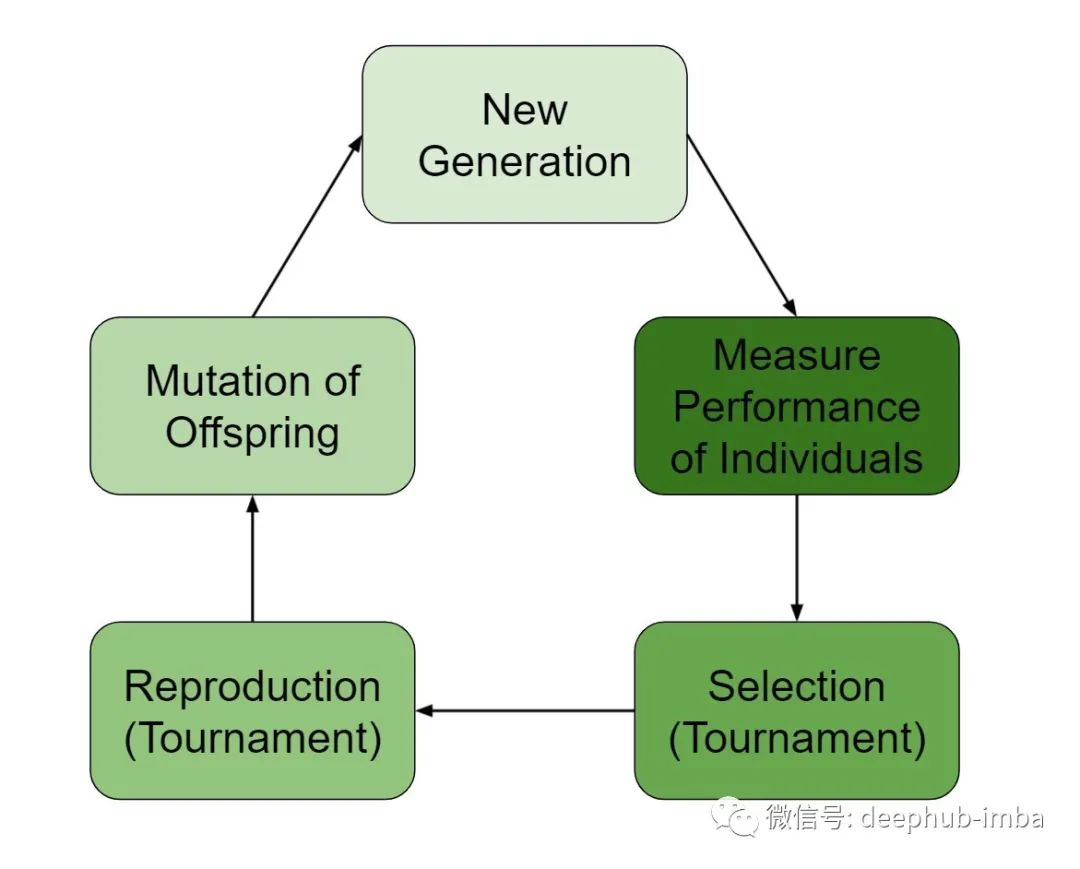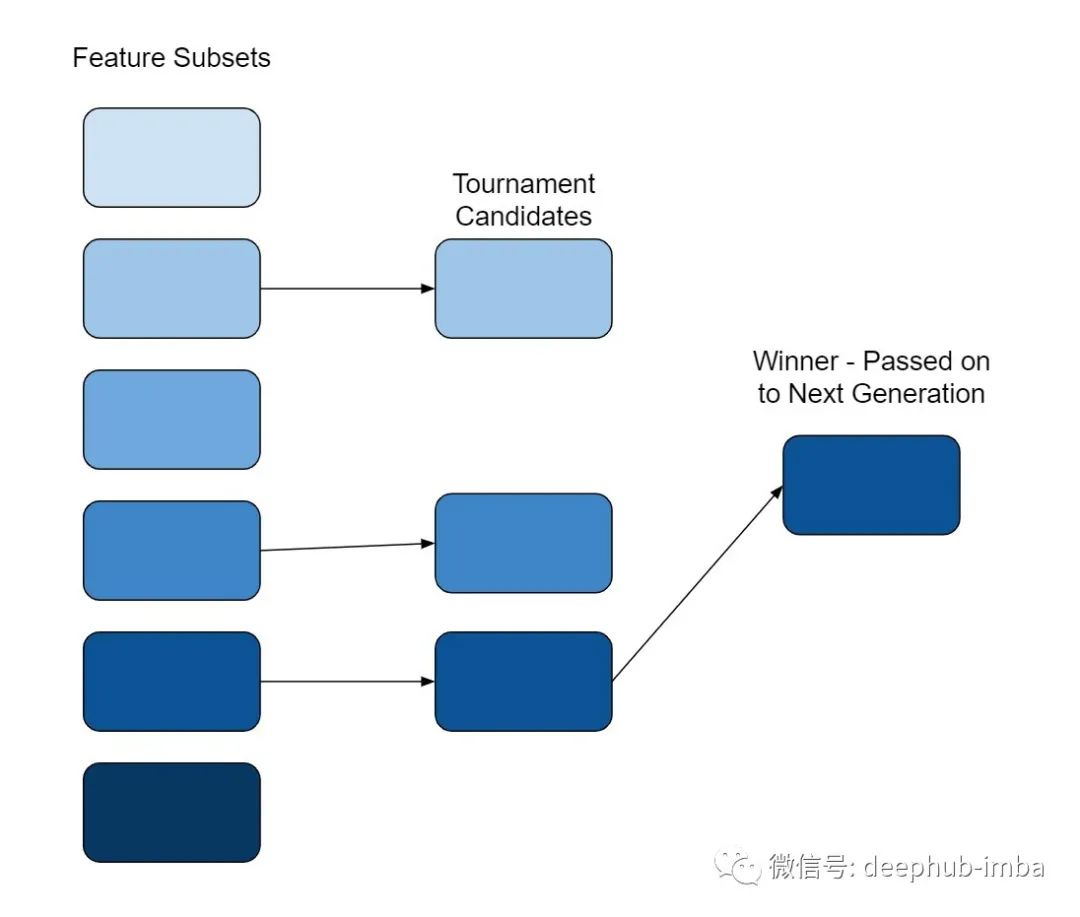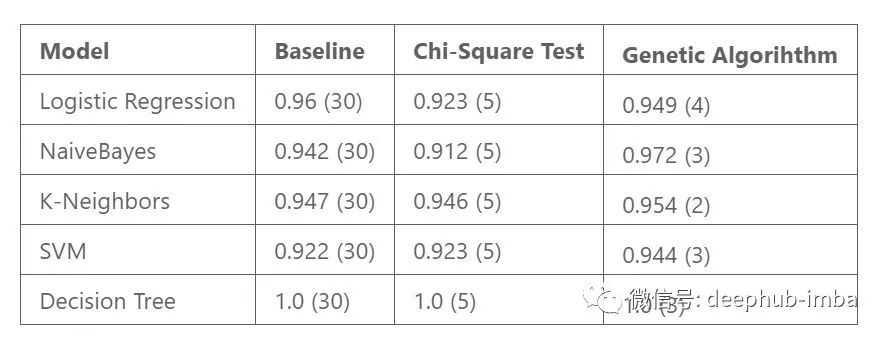# 基于遗传算法的特征选择:通过自然选择过程确定最优特征集

2021/09/12 09:24

## 遗传算法## 特征选择

1. 产生初始种群

2. 对种群中的每个成员进行评分

3. 通过竞赛选择子集进行繁殖

4. 选择要传递的遗传物质（特征）

5. 应用突变

6. 以上步骤重复多次，每一次成为一代（generation）

## 实际操作

from sklearn.datasets import load_breast_cancerfrom genetic_selection import GeneticSelectionCVfrom sklearn.tree import DecisionTreeClassifierimport pandas as pdimport numpy as npdata = load_breast_cancer()df = pd.DataFrame(data.data, columns=data.feature_names)df['target'] = data.targetX = df.drop(['target'], axis=1)y = df['target'].astype(float)estimator = DecisionTreeClassifier()model = GeneticSelectionCV(    estimator, cv=5, verbose=0,    scoring="accuracy", max_features=5,    n_population=100, crossover_proba=0.5,    mutation_proba=0.2, n_generations=50,    crossover_independent_proba=0.5,    mutation_independent_proba=0.04,    tournament_size=3, n_gen_no_change=10,    caching=True, n_jobs=-1)model = model.fit(X, y)print('Features:', X.columns[model.support_])

GeneticSelectionCV## 总结点个 在看 你最好看！0 评论
0 收藏
0